NEET  >  Laws of Thermodynamics & Heat Engine

# Laws of Thermodynamics & Heat Engine - Physics Class 11 - NEET

Zeroth Law of Thermodynamics:

According to this law, two systems in thermal equilibrium with a third system separately are in thermal equilibrium with each other. Thus, if A and B are separately in equilibrium with C, that is if TA = TC and TB = TC, then this implies that TA = TB i.e., the systems A and B are also in thermal equilibrium.

First Law of Thermodynamics:

Heat given to a thermodynamic system (ΔQ) is partially utilized in doing work (ΔW) against the surrounding and the remaining part increases the internal energy (ΔU) of the system.

Therefore, ΔQ = ΔU + ΔW

First law of thermodynamics is a restatement of the principle conservation of energy.

In an isothermal process, change in internal energy is zero (ΔU = 0).

Therefore, ΔQ = ΔW

In an adiabatic process, no exchange of heat takes place, i.e., Δθ = O.

Therefore, ΔU = – ΔW

In an adiabatic process, if gas expands, its internal energy and hence, temperature decreases and vice-versa.

In an isochoric process, work done is zero, i.e., ΔW = 0, therefore

ΔQ = ΔU

Thermodynamic Processes:

A thermodynamical process is said to take place when some changes occur in the state of a thermodynamic system i.e., the thermodynamic parameters of the system change with time.

(i) Isothermal Process:

A process taking place in a thermodynamic system at constant temperature is called an isothermal process.

Isothermal processes are very slow processes.

These process follows Boyle’s law, according to which

pV = constant

From dU = nCvdT as dT = 0 so dU = 0, i.e., internal energy is constant.

From first law of thermodynamics, dQ = dW, i.e., heat given to the system is equal to the work done by system surroundings.

Work done W = 2.3026μRT l0g10(Vf / Vi) = 2.3026μRT l0g10(pi / pf)

where, μ = number of moles, R = ideal gas constant, T = absolute temperature and Vi Vand Pi, Pf are initial volumes and pressures.

After differentiating P V = constant, we have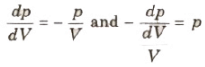i.e., bulk modulus of gas in isothermal process, β = p.

P – V curve for this persons is a rectangular hyperbola

Examples:

(a) Melting process is an isothermal change, because temperature of a substance remains constant during melting.

(b) Boiling process is also an isothermal operation.

A process taking place in a thermodynamic system for which there is no exchange of heat between the system and its surroundings.

Adiabatic processes are very fast processes.

These process follows Poisson’s law, according to which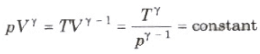From dQ = nCdT, Cadi = 0 as dQ = 0, i.e., molar heat capacity for adiabatic process is zero.

From first law, dU = – dW, i.e., work done by the system is equal to decrease in internal energy. When a system expands adiabatically, work done is positive and hence internal energy decrease, i.e., the system cools down and vice-versa.

Work done in an adiabatic process is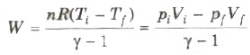where Ti and Tf are initial and final temperatures. Examples

(a) Sudden compression or expansion of a gas in a container with perfectly non-conducting wall.

(b) Sudden bursting of the tube of a bicycle tyre.

(c) Propagation of sound waves in air and other gases.

(iii) Isobaric Process

A process taking place in a thermodynamic system at constant pressure is called an isobaric process.

Molar heat capacity of the process is Cp and dQ = nCpdT.

Internal energy dU = nCv dT

From the first law of thermodynamics
dQ = dU + dW
dW = pdV = nRdT

Process equation is V / T = constant.

p- V curve is a straight line parallel to volume axis.

(iv) Isochoric Process

A process taking place in a thermodynamics system at constant volume is called an isochoric process.

dQ = nCvdT, molar heat capacity for isochoric process is Cv.

Volume is constant, so dW = 0,

Process equation is p / T = constant

p- V curve is a straight line parallel to pressure axis.

(v) Cyclic Process

When a thermodynamic system returns to the initial state after passing through several states, then it is called cyclic process.
Efficiency of the cycle is given by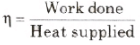Work done by the cycle can be computed from area enclosed cycle on p- V curve.

The graph drawn between the pressure p and the volume V of a given mass of a gas for an isothermal process is called isothermal curve and for an adiabatic process it is called adiabatic curve .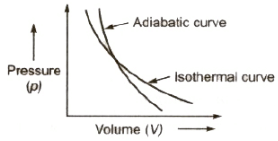Fig: Isothermal vs adiabatic curve

The slope of the adiabatic curve

= γ x the slope of the isothermal curve

Volume Elasticities of Gases:

There are two types of volume elasticities of gases:

(i) Isothermal modulus of elasticity ES = p

(ii) Adiabatic modulus of elasticity ET = γ p

Ratio between isothermal and adiabatic modulus

ES / ET = γ = Cp / CV

where Cp and Cv are specific heats of gas at constant pressure and at constant volume.

For an isothermal process Δt = 0, therefore specific heat,

c = Δ θ / m Δt = &;

For an adiabatic process 119= 0, therefore specific heat,

c = 0 / m Δt = 0

Second Law of Thermodynamics:

The second law of thermodynamics gives a fundamental limitation to the efficiency of a heat engine and the coefficient of performance of a refrigerator. It says that efficiency of a heat engine can never be unity (or 100%). This implies that heat released to the cold reservoir can never be made zero.

Kelvin’s Statement:

It is impossible to obtain a continuous supply of work from a body by cooling it to a temperature below the coldest of its surroundings.

Clausius’ Statement:

It is impossible to transfer heat from a lower temperature body to a higher temperature body without use of an external agency.

Planck’s Statement:

It is impossible to construct a heat engine that will convert heat completely into work.

All these statements are equivalent as one can be obtained from the other.

Entropy:

Entropy is a physical quantity that remains constant during a reversible adiabatic change.

Change in entropy is given by dS = δQ / T

Where, δQ = heat supplied to the system

and T = absolute temperature.

Entropy of a system never decreases, i.e., dS ≥ o.

Entropy of a system increases in an irreversible process

Heat Engine:

A heat energy engine is a device which converts heat energy into mechanical energy.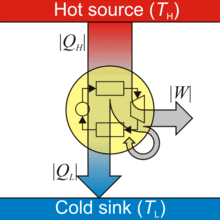Fig: Heat Engine

A heat engine consists of three parts:

(i) Source of heat at higher temperature

(ii) Working substance

(iii) Sink of heat at lower temperature

Thermal efficiency of a heat engine is given by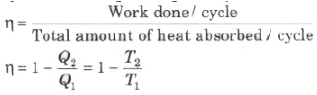where Q1 is heat absorbed from the source,

Q2 is heat rejected to the sink and T1 and T2 are temperatures of source and sink.

Heat engine are of two types:

(i) External Combustion Engine: In this engine fuel is burnt a chamber outside the main body of the engine. e.g., steam engine. In practical life thermal efficiency of a steam engine varies from 12% to 16%.

(ii) Internal Combustion Engine: In this engine, fuel is burnt inside the main body of the engine. e.g., petrol and diesel engine. In practical life thermal efficiency of a petrol engine is 26% and a diesel engine is 40%.

The document Laws of Thermodynamics & Heat Engine | Physics Class 11 - NEET is a part of the NEET Course Physics Class 11.
All you need of NEET at this link: NEET

## FAQs on Laws of Thermodynamics & Heat Engine - Physics Class 11 - NEET

 1. What are the laws of thermodynamics?Ans. The laws of thermodynamics are fundamental principles that govern the behavior of energy in a system. There are four laws, namely the zeroth law, the first law, the second law, and the third law. These laws explain how energy is transferred and transformed in various processes.
 2. What is a heat engine?Ans. A heat engine is a device that converts thermal energy into mechanical work. It operates based on the principles of thermodynamics, specifically the second law. Heat engines typically involve the cyclic transfer of heat from a high-temperature source to a low-temperature sink, producing useful work in the process.
 3. How does the first law of thermodynamics relate to heat engines?Ans. The first law of thermodynamics, also known as the law of energy conservation, states that energy cannot be created or destroyed; it can only be transferred or transformed. In the context of heat engines, the first law implies that the net work output of the engine is equal to the difference between the heat input and the heat rejected to the surroundings.
 4. What is the second law of thermodynamics and its significance for heat engines?Ans. The second law of thermodynamics states that the entropy of an isolated system always increases over time. In the context of heat engines, this law imposes limitations on their efficiency. It implies that no heat engine can have 100% efficiency, as some energy will always be lost to the surroundings as waste heat.
 5. What is the significance of the third law of thermodynamics in relation to heat engines?Ans. The third law of thermodynamics states that as the temperature of a system approaches absolute zero, the entropy of the system approaches a minimum value. While the third law may not have a direct impact on the operation of heat engines, it helps establish the concept of absolute zero temperature as a reference point for thermodynamic calculations.

## Physics Class 11

130 videos|483 docs|210 tests

## Physics Class 11

130 videos|483 docs|210 tests

### How to Prepare for NEET

Read our guide to prepare for NEET which is created by Toppers & the best Teachers
Signup to see your scores go up within 7 days! Learn & Practice with 1000+ FREE Notes, Videos & Tests.
10M+ students study on EduRev
Track your progress, build streaks, highlight & save important lessons and more!(Scan QR code)
Related Searches

,

,

,

,

,

,

,

,

,

,

,

,

,

,

,

,

,

,

,

,

,

;